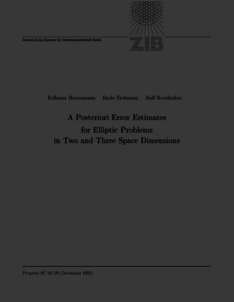Repository: Freie Universität Berlin, Math Department

# A posteriori error estimates for elliptic problems in two and three space dimensions

Bornemann, F. A. and Erdmann, B. and Kornhuber, R. (1996) A posteriori error estimates for elliptic problems in two and three space dimensions. SIAM Journal on Numerical Analysis, 33 (3). pp. 1188-1204. ISSN 0036-1429Preview

271kB

Official URL: http://dx.doi.org/10.1137/0733059

## Abstract

Let $u \in H$ be the exact solution of a given selfadjoint elliptic boundary value problem, which is approximated by some $\tilde u \in \mathcal{S}$, $\mathcal{S}$ being a suitable finite-element space. Efficient and reliable a posteriors estimates of the error $\| {u - \tilde u} \|$, measuring the (local) quality of $\tilde u$, play a crucial role in termination criteria and in the adaptive refinement of the underlying mesh. A well-known class of error estimates can be derived systematically by localizing the discretized defect problem by using domain decomposition techniques. In this paper, we provide a guideline for the theoretical analysis of such error estimates. We further clarify the relation to other concepts. Our analysis leads to new error estimates, which are specially suited to three space dimensions. The theoretical results are illustrated by numerical computations.

Item Type: Article adaptive finite-element methods, a posteriors error estimates Mathematical and Computer Sciences > Mathematics > Numerical Analysis Department of Mathematics and Computer Science > Institute of Mathematics 1910 Ekaterina Engel 21 Jun 2016 19:48 03 Mar 2017 14:42

Repository Staff Only: item control page